# Bi-unitary multiperfect numbers, IV(b)

Pentti Haukkanen and Varanasi Sitaramaiah
Notes on Number Theory and Discrete Mathematics
Print ISSN 1310–5132, Online ISSN 2367–8275
Volume 27, 2021, Number 1, Pages 45—69
DOI: 10.7546/nntdm.2021.27.1.45-69

## Details

### Authors and affiliations

Pentti HaukkanenFaculty of Information Technology and Communication Sciences
FI-33014 Tampere University, Finland

Varanasi Sitaramaiah
1/194e, Poola Subbaiah Street, Taluk Office Road, Markapur
Prakasam District, Andhra Pradesh, 523316 India

### Abstract

A divisor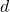of a positive integer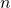is called a unitary divisor if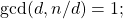andis called a bi-unitary divisor ofif the greatest common unitary divisor ofand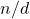is unity. The concept of a bi-unitary divisor is due to D. Surynarayana (1972). Letdenote the sum of the bi-unitary divisors of. A positive integeris called a bi-unitary multiperfect number iffor some. For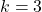we obtain the bi-unitary triperfect numbers.

Peter Hagis (1987) proved that there are no odd bi-unitary multiperfect numbers. The present paper is part IV(b) in a series of papers on even bi-unitary multiperfect numbers. In parts I, II and III we considered bi-unitary triperfect numbers of the form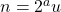, where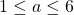andis odd. In part IV(a) we solved partly the case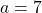. We proved that ifis a bi-unitary triperfect number of the form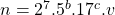, where, then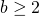. We then solved completely the case. In the present paper we give some partial results concerning the case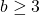under the assumption### Keywords

• Perfect numbers
• Triperfect numbers
• Multiperfect numbers
• Bi-unitary analogues

• 11A25

### References

1. Hagis, P., Jr. (1987). Bi-unitary amicable and multiperfect numbers, Fibonacci Quart., 25(2), 144–150.
2. Haukkanen, P. & Sitaramaiah, V. (2020). Bi-unitary multiperfect numbers, I, Notes on Number Theory Discrete Mathematics, 26 (1), 93–171.
3. Haukkanen, P., & Sitaramaiah, V. (2020). Bi-unitary multiperfect numbers, II. Notes on Number Theory and Discrete Mathematics, 26(2), 1–26.
4. Haukkanen, P., & Sitaramaiah, V. (2020). Bi-unitary multiperfect numbers, III. Notes on Number Theory and Discrete Mathematics, 26(3), 33–67.
5. Haukkanen, P., & Sitaramaiah, V. (2020). Bi-unitary multiperfect numbers, IV(a). Notes on Number Theory and Discrete Mathematics, 26(4), 2–32.
6. Sándor, J. & Crstici, P. (2004). Handbook of Number Theory II, Kluwer Academic.
7. Suryanarayana, D. (1972). The number of bi-unitary divisors of an integer, in The Theory of Arithmetic Functions, Lecture Notes in Mathematics 251: 273–282, New York, Springer–Verlag.
8. Wall, C. R. (1972). Bi-unitary perfect numbers, Proc. Amer. Math. Soc., 33(1), 39–42.

## Cite this paper

Haukkanen, P., & Sitaramaiah, V. (2021). Bi-unitary multiperfect numbers, IV(b). Notes on Number Theory and Discrete Mathematics, 27(1), 4569, doi: 10.7546/nntdm.2021.27.1.45-69.